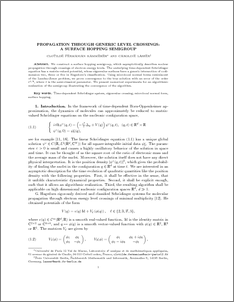Repository: Freie Universität Berlin, Math Department

# Propagation through Generic Level Crossings: A Surface Hopping Semigroup

Fermanian Kammerer, C. and Lasser, C. (2008) Propagation through Generic Level Crossings: A Surface Hopping Semigroup. J. Math. Anal., 40 (1). pp. 103-133.Preview

361kB

Official URL: http://dx.doi.org/10.1137/070686810

## Abstract

We construct a surface hopping semigroup, which asymptotically describes nuclear propagation through crossings of electron energy levels. The underlying time-dependent Schrödinger equation has a matrix-valued potential, whose eigenvalue surfaces have a generic intersection of codimension two, three, or five in Hagedorn's classification. Using microlocal normal forms reminiscent of the Landau–Zener problem, we prove convergence to the true solution with an error of the order $\varepsilon^{1/8}$, where $\varepsilon$ is the semiclassical parameter. We present numerical experiments for an algorithmic realization of the semigroup illustrating the convergence of the algorithm.

Item Type: Article Physical Sciences > Physics > Mathematical & Theoretical Physics > Quantum Mechanics Department of Mathematics and Computer Science > Institute of Mathematics 903 Burkhard Schmidt 29 Apr 2010 08:02 03 Mar 2017 14:40

Repository Staff Only: item control page